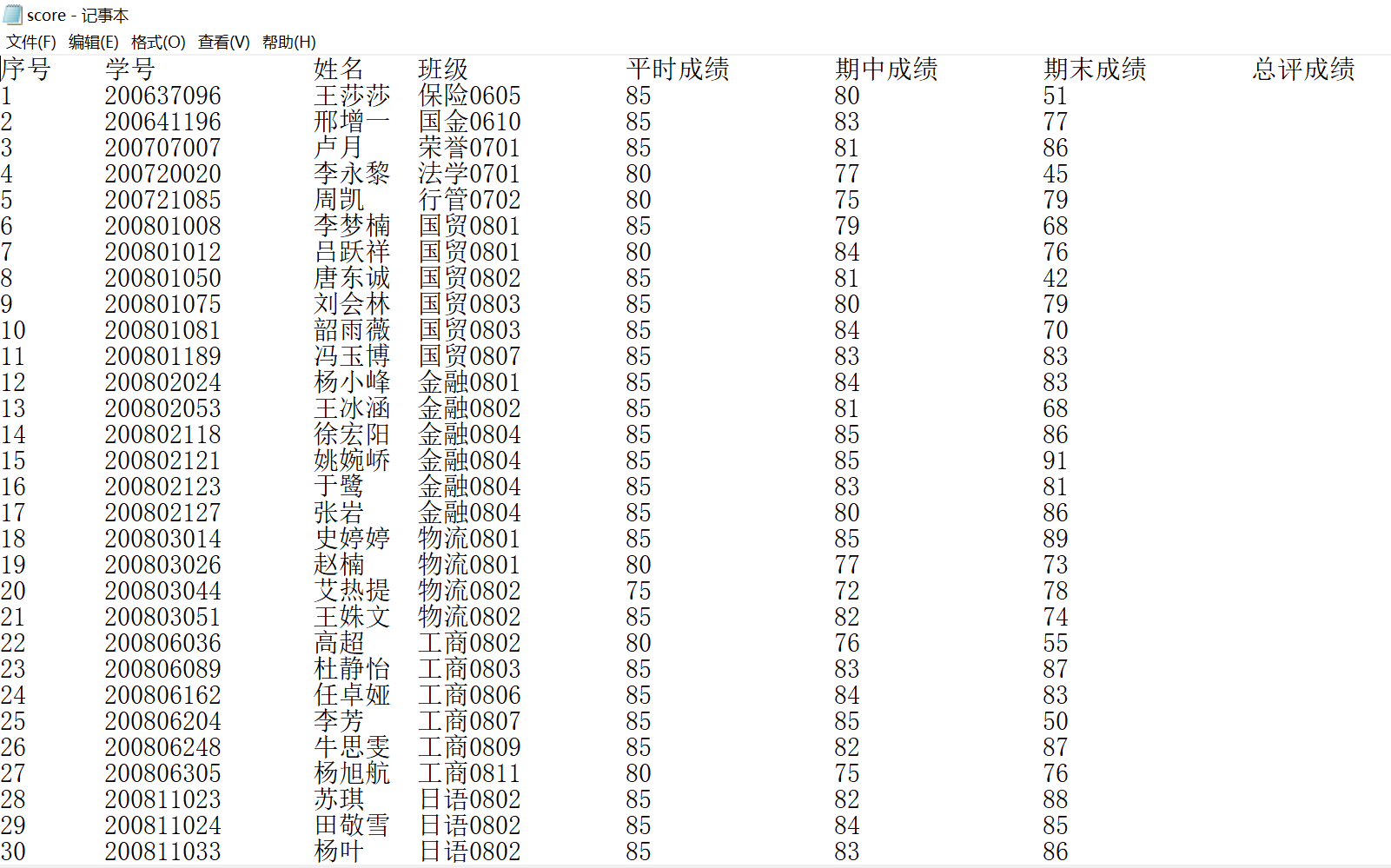struct StudentScore{

int serialNum;//序号

int id;//学号

string name;//姓名

string major;//专业

float regularScore;//平时成绩

float midtermScore;//期中成绩

float examScore;//期末成绩 float finalScore;//总评成绩

};

void printScore(StudentScore *stu); //输出到屏幕

void parseScore(StudentScore *s, string str); //解析一行文本

bool readFromFile(StudentScore *s, int count, string inputFileName); /
/从文件读取

void calculateMajor (StudentScore *s);//统计班上各专业人数并写入文件

void calculateFinalScore (StudentScore *s, int count); //计算总评成绩

void sortScoreDesc(StudentScore *s, int count); //排序函数，总成绩降序

//排序可以链表也可以数组

void writeToFile(StudentScore *s, int count,string outputFileName); //2个回答

major.txt：统计班上各专业人数
result.txt：排序函数，总成绩降序

``````#include <iostream>
#include <string.h>
#include <fstream>
#include <vector>
#include <algorithm>

using namespace std;

struct StudentScore{
int serialNum;//序号
int id;//学号
string name;//姓名
string major;//专业
float regularScore;//平时成绩
float midtermScore;//期中成绩
float examScore;//期末成绩
float finalScore;//总评成绩
};

StudentScore stu;
int totalcount = 0;

//输出到屏幕
void printScore(StudentScore *stu)
{
cout << "序号    :" << stu->serialNum << endl;
cout << "学号    :" << stu->id << endl;
cout << "姓名    :" << stu->name << endl;
cout << "专业    :" << stu->major << endl;
cout << "平时成绩 :" << stu->regularScore << endl;
cout << "期中成绩 :" << stu->midtermScore << endl;
cout << "期末成绩 :" << stu->examScore << endl;
cout << "总评成绩 :" << stu->finalScore << endl;
}

//解析一行文本
void parseScore(StudentScore *s, string str)
{
//1     200637096       王莎莎      保险0605        85      80      51
if(s != NULL )
{
int flag = 0;
int flag1 = 0;
string temp ;

//
for(auto iter = str.begin(); iter != str.end(); ++iter)
{
if((*iter != ' ') && (*iter != '\t'))
{
//有效数据标志
flag1 = 1;
temp += *iter;
} else {
if(!flag1)
{
continue;
}

//数据内容
switch(flag)
{
case 0:
s->serialNum = stoi(temp);
flag++;
break;
case 1:
s->id = stoi(temp);
flag++;
break;
case 2:
s->name = temp;
flag++;
break;
case 3:
s->major = temp;
flag++;
break;
case 4:
s->regularScore = stof(temp);
flag++;
break;
case 5:
s->midtermScore = stof(temp);
flag++;
break;
case 6:
s->examScore = stof(temp);
flag++;
break;
default:
break;
}
temp.clear();
flag1 = 0;
}
}
s->examScore = stof(temp);

// printScore(s);
}

}

//从文件读取
bool readFromFile(StudentScore *s, int count, string inputFileName)
{
int i = 0;
ifstream ifile;
string line;
ifile.open(inputFileName);

getline(ifile, line);
// cout << line << endl;

while(ifile)
{
getline(ifile, line);
//防止重复读取最后一行
if(ifile.peek() == '\n')
break;

parseScore(&s[i++], line);

totalcount++;

if(i >= count)
{
return false;;
}
}

return true;
}

struct MajorInfo {
string major;
int count;
};

//统计班上各专业人数并写入文件
void calculateMajor (StudentScore *s, int count)
{
int flag = 1;
vector<MajorInfo> vm;
ofstream fout("major.txt");

for(int i=0 ; i< count; i++)
{
flag = 0;
for(auto iter = vm.begin(); iter != vm.end(); iter++)
{
if(s[i].major.compare(iter->major) == 0)
{
flag = 1;
iter->count++;
}
}

if(!flag)
{
MajorInfo temp;

temp.major = s[i].major;
temp.count = 1;

vm.push_back(temp);
}
}
fout << "班级专业\t\t数量" << endl;
for(auto iter = vm.begin(); iter != vm.end(); iter++)
{
fout << iter->major << "\t\t" << iter->count << endl;
}

fout.close();
}

//计算总评成绩
void calculateFinalScore (StudentScore *s, int count)
{
for(int i = 0; i < count; i++)
{
s[i].finalScore = s[i].regularScore * 0.1 + s[i].midtermScore * 0.3 + s[i].examScore * 0.6;
}
}

bool myfunction(StudentScore a, StudentScore b)
{
return (a.finalScore < b.finalScore);
}

//排序函数，总成绩降序排，
void sortScoreDesc(StudentScore *s, int count)
{
sort(&s, &s[count], myfunction);
}

//排序可以链表也可以数组
void writeToFile(StudentScore *s, int count,string outputFileName)
{
ofstream fout(outputFileName);

fout <<"序号\t\t学号\t\t姓名\t\t班级\t\t平时成绩\t\t期中成绩\t\t期末成绩\t\t总评成绩"<< endl;
for(int i=0; i< count; i++)
{
// printScore(&s[i]);
fout << s[i].serialNum << "\t\t" << s[i].id  << "\t" << s[i].name << "\t\t" \
<< s[i].major << "\t" << s[i].regularScore << "\t\t\t" << s[i].midtermScore << "\t\t\t" \
<< s[i].examScore << "\t\t\t" << s[i].finalScore << endl;
}

fout.close();
}

int main(int argc, char *argv[])
{
bool ret;

//string str = "1     200637096       王莎莎      保险0605        85      80      51";
//parseScore(&stu, str);
if(ret == false)
{
return -1;
}

calculateMajor(stu, totalcount);

calculateFinalScore(stu, totalcount);

sortScoreDesc(stu, totalcount);

writeToFile(stu, totalcount, "result.txt");

return 0;
}

``````Python+OpenCV计算机视觉

Python+OpenCV计算机视觉

Problem Description 转眼又到了一年的年末，Lele又一次迎来了期末考试。虽然说每年都要考试，不过今年的这场考试对Lele来说却意义重大。 因为经济原因，如果今年没有排在班级前几名，而拿不到奖学金的话，家里便无力再供他继续读书。而且家里帮他都想好出路了——回家种田！！ 虽说Lele心里有一百个不愿意，不过父母的话不能不听。 忐忑不安地考完试，Lele拿到了全班的成绩单，这张成绩单是按学号顺序排好的。Lele很想知道班里到底有多少人分数比他高，现在就请你帮帮他，帮他数一下到底有多少人的分数比他高吧。 Input 数据的第一行有一个正整数T，表示测试的组数。接下来有T组测试。 每组数据包括两行。 第一行有两个正整数N K(0<N<1000,0<K<=N),分别表示成绩单上一共的学生数目，和Lele的学号。 第二行有N个整数Xi（0<=Xi<=100）分别表示各个学生的成绩，以学号递增顺序给出，第一个学生学号为1。 Output 对于每组数据，请在一行里输出班里一共有多少个学生成绩高于Lele Sample Input 1 3 2 81 72 63 Sample Output 1

c语言课程设计——数据统计程序

C语言 学生成绩管理系统

c语言程序 ———栈的实现

#include<stdio.h> #include<stdlib.h> #define STACK_INIT_SIZE 10 #define STACKINCREMENT 2 struct Stack { char *base; char *top; int stacksize; }; struct Stack *InitStack(struct Stack *S) //创建空栈 { S->base=(char *)malloc(STACK_INIT_SIZE * sizeof(char)); if(!S->base) {printf("error!"); return 0;} S->top=S->base; S->stacksize=STACK_INIT_SIZE ; return S; } struct Stack *Push(struct Stack *S,char e) //向栈中插入元素 { if(S->top-S->base==S->stacksize) { S->base=(char *)realloc(S->base,(S->stacksize+STACKINCREMENT * sizeof(char))); if(!S->base) {printf("分配空间失败"); return 0;} S->top=S->base+S->stacksize; S->stacksize+=STACKINCREMENT; } *(S->top)=e; S->top++; return S; } void Pop(struct Stack *S,char e) //删除栈顶元素，并且返回其值 { if(S->top==S->base) {printf("栈为空，无法删除栈顶元素"); return 0;} e=*(--S->top); printf("删除的栈顶元素为："); printf("%c\n",e); } int main() { struct Stack *S; int e; char i; S=(struct Stack *)malloc(STACK_INIT_SIZE * sizeof(char)); if( !S->base) printf("error!"); InitStack(S); printf("输入要插入的元素e:"); scanf("%c",&e); Push(S,&e); Pop(S,&i); return 0; } 以上是我写的c语言程序，运行的时候不管输入什么，输出的都是x，求哪位大神讲解下为什么，实在是百思不得其解

C语言——统计文件中出现最多的前5个字母

1) 定义一个矩形（Rectangle）类，该类代表了一个矩形。可以定义不同的矩形，并对矩形进行如下运算：  移动矩形  判断一个点是否在矩形内部  求两个矩形合并后的矩形，通过函数返回值返回代表合并后矩形的新建立 的矩形对象  求两个矩形交集，通过函数返回值返回代表两个矩形交集的一个新建立的 矩形对象 . 点不在矩形内部 合并后的矩形 两个矩形的交集 设计提示 矩形类提示如下： (1) 左上角坐标（x, y,）和矩形的宽度width、高度height可以描绘一个矩形 。 (2) 由于要判断点是否在矩形内，所以判断函数(isInside)应该作为该类的方 法，同样合并矩形（unionWith）和求两个矩形的交集（intersection）也分 别是方法。 (3) 可以定义多个构造函数，第一个是无参构造函数Rectangle，此时默认为 左上角和右下角的坐标都是（0，0），实际是一个点。第二个有4个参数Rect angle (double x, double y, double width, double height)，分别代表左上 角坐标、宽度和高度。第三个有两个参数Rect(double width, double heigh t)，认为左上角和右下角坐标分别是（0,0）和（width, height）。 测试代码提示如下： （1） 定义多个矩形对象和点坐标变量 （2） 调用对象方法isInside判断一个点是否在矩形内并打印合并后的结果 （3） 调用unionWith合并矩形并打印合并后的结果 （4） 调用intersection求矩形的交集并打印合并后的结果

![图片说明](https://img-ask.csdn.net/upload/201906/04/1559631967_426562.jpg) 使用C++并采用蒙特卡洛方法求图1中阴影部分的面积 蒙特卡洛方法举例：用蒙特卡罗方法计算圆周率π。 1. 正方形内部有一个相切的圆，如图2所示。 图2 与圆型相切的正方形 2. 圆形的面积与正方形的面积之比是π/4，面积之比如式(1)。 (1) 3. 现在，在这个正方形内部，随机产生10000个点（即10000个坐标对 (x, y)），计算它们与中心点的距离，从而判断是否落在圆的内部，如图3所示。 图3 随机产生正方形内部的点 4. 如果这些点均匀分布，那么圆内的点应该占到所有点的 π/4，因此将这个比值乘以4，就是π的值。通过随机模拟30000个点，π的估算值与真实值相差0.07%。

``` #include<stdio.h> #include<math.h> #include<stdlib.h> #include<string.h> int main() { int N,n,i; char t,s; scanf("%d",&N); while(N--) { gets(t); scanf("%d",&n); for(i=0;i<n;i++) s[i] = t[i]; s[n] = '\0'; puts(s); } } ``` 这样写为什么程序运行的时候会出错啊/(ㄒoㄒ)/~~

oracle 触发器。当表中期中成绩字段或期末成绩字段任一个改变时 总成绩随之改变。

C++程序——数组与函数

1、定义Circle类和Square类，Circle类的成员变量为半径和面积，Square类的成员变量为边长和面积。 2、生成Circle类对象和Square类对象，并让Square类对象内接Circle类对象。 3、给定Square类对象的边长，调用各自的计算面积的成员函数，分别计算各自的面积。

2019 Python开发者日-培训

2019 Python开发者日-培训

150讲轻松搞定Python网络爬虫

150讲轻松搞定Python网络爬虫

YOLOv3目标检测实战：训练自己的数据集

YOLOv3目标检测实战：训练自己的数据集

java后台+微信小程序 实现完整的点餐系统

java后台+微信小程序 实现完整的点餐系统

2019 AI开发者大会

2019 AI开发者大会

4小时玩转微信小程序——基础入门与微信支付实战

4小时玩转微信小程序——基础入门与微信支付实战

Git 实用技巧

Git 实用技巧

Python数据清洗实战入门

Python数据清洗实战入门

MySQL数据库从入门到实战应用

MySQL数据库从入门到实战应用

.net core快速开发框架

.net core快速开发框架

Python数据挖掘简易入门

Python数据挖掘简易入门

Windows版YOLOv4目标检测实战：训练自己的数据集

Windows版YOLOv4目标检测实战：训练自己的数据集

HoloLens2开发入门教程

HoloLens2开发入门教程

Java8零基础入门视频教程

Java8零基础入门视频教程

Python可以这样学（第一季：Python内功修炼）

Python可以这样学（第一季：Python内功修炼）